# Selina Solutions Concise Maths Class 7 Chapter 2: Rational Numbers Exercise 2A

Selina Solutions Concise Maths Class 7 Chapter 2 Rational Numbers Exercise 2A covers the introduction to the basic concepts of rational numbers. In a subject like Mathematics, it is necessary for the students to understand the concepts thoroughly. After proper understanding, students can solve the exercise wise problems given in the textbook. If students are not able to answer any difficult problems, they can refer to the solutions PDF designed by subject matter experts. Solving the problems using Selina Solutions Concise Maths Class 7 Chapter 2 Rational Numbers Exercise 2A free PDF improves confidence among students, in facing the exam without fear.

## Selina Solutions Concise Maths Class 7 Chapter 2: Rational Numbers Exercise 2A Download PDF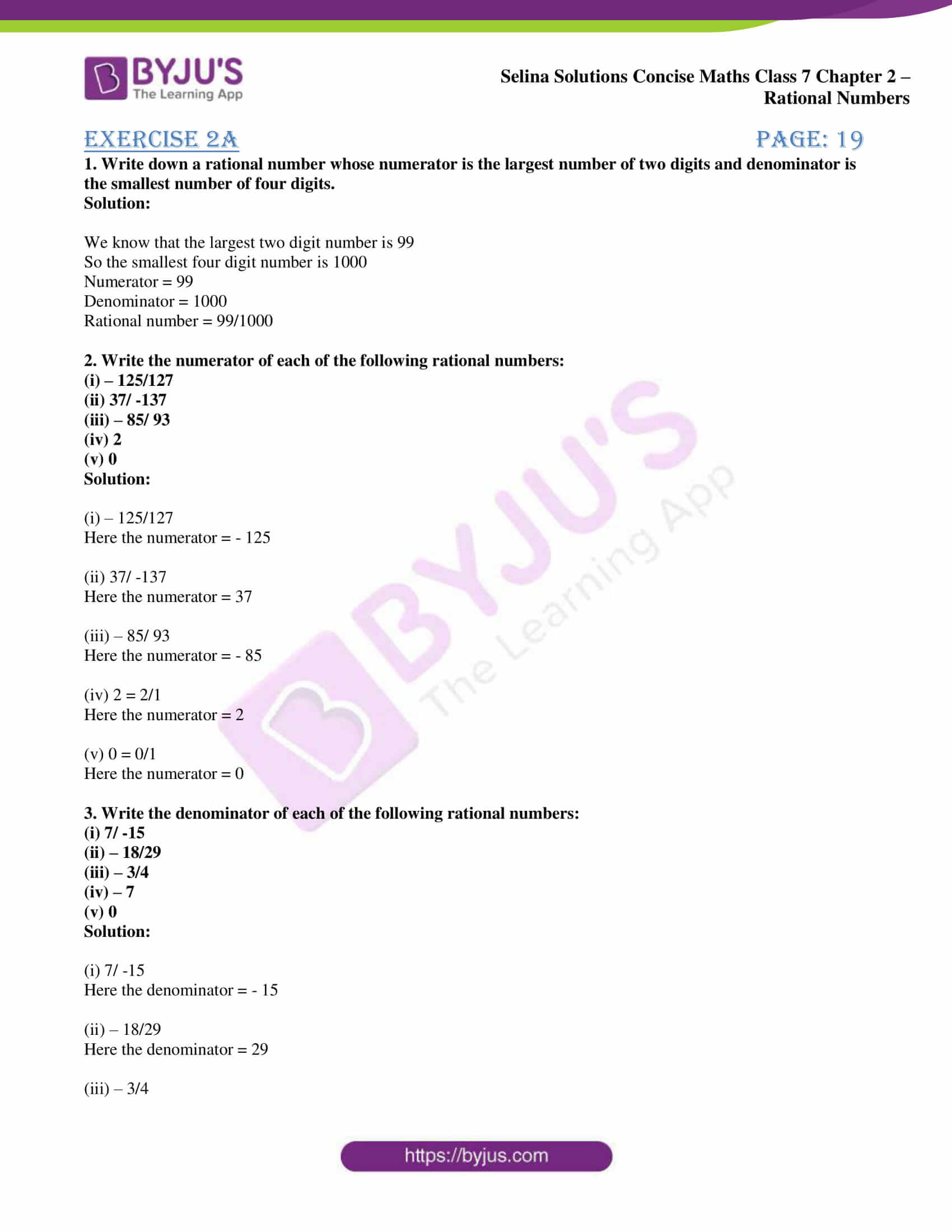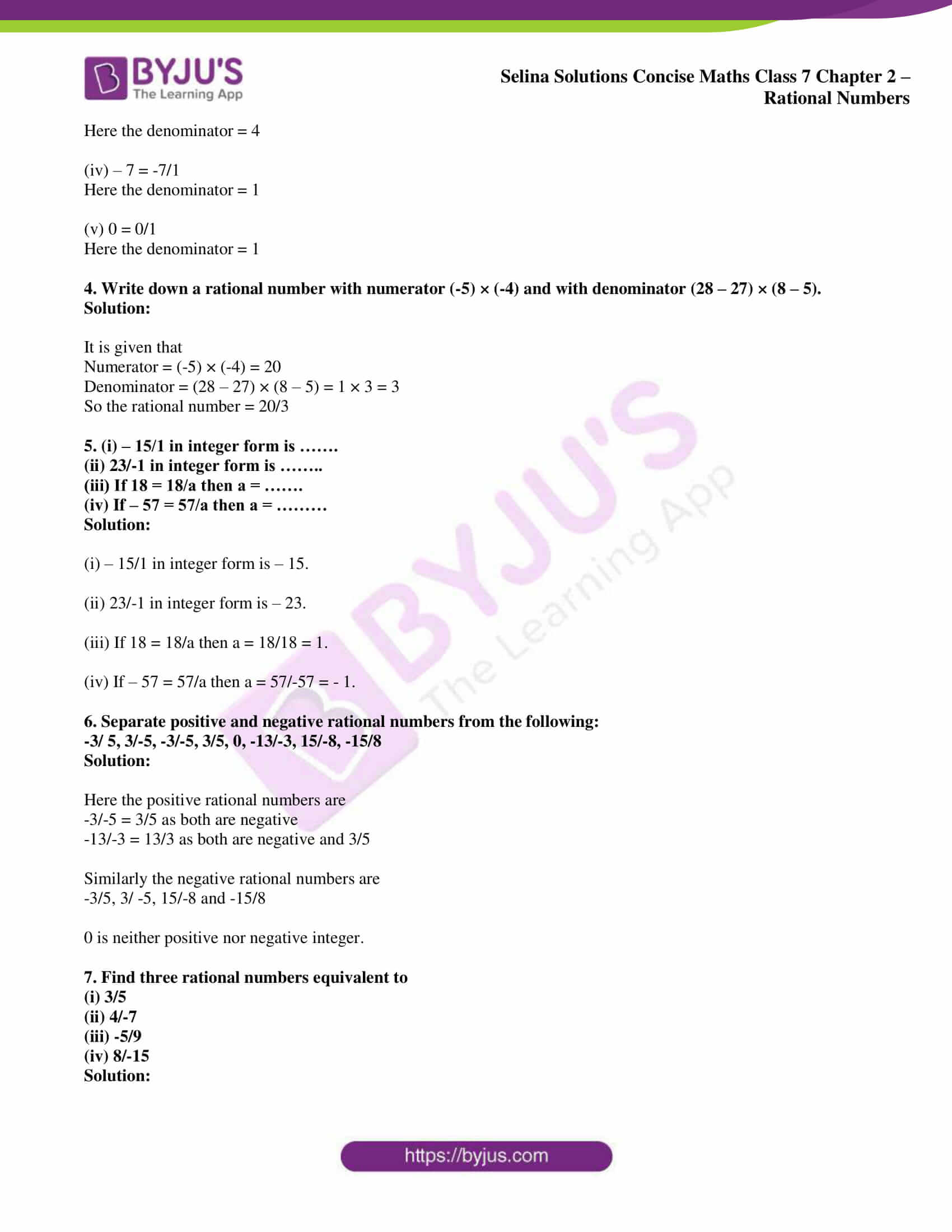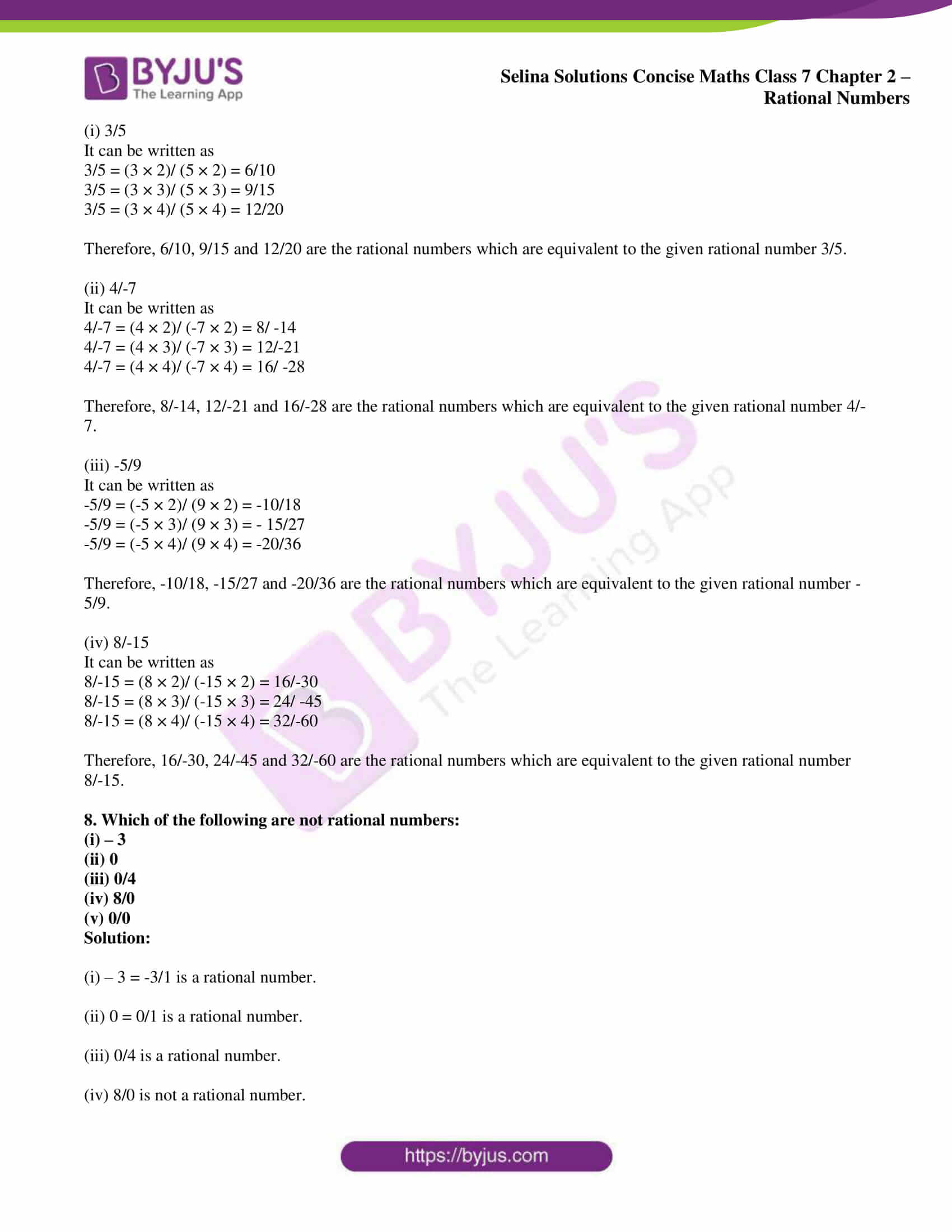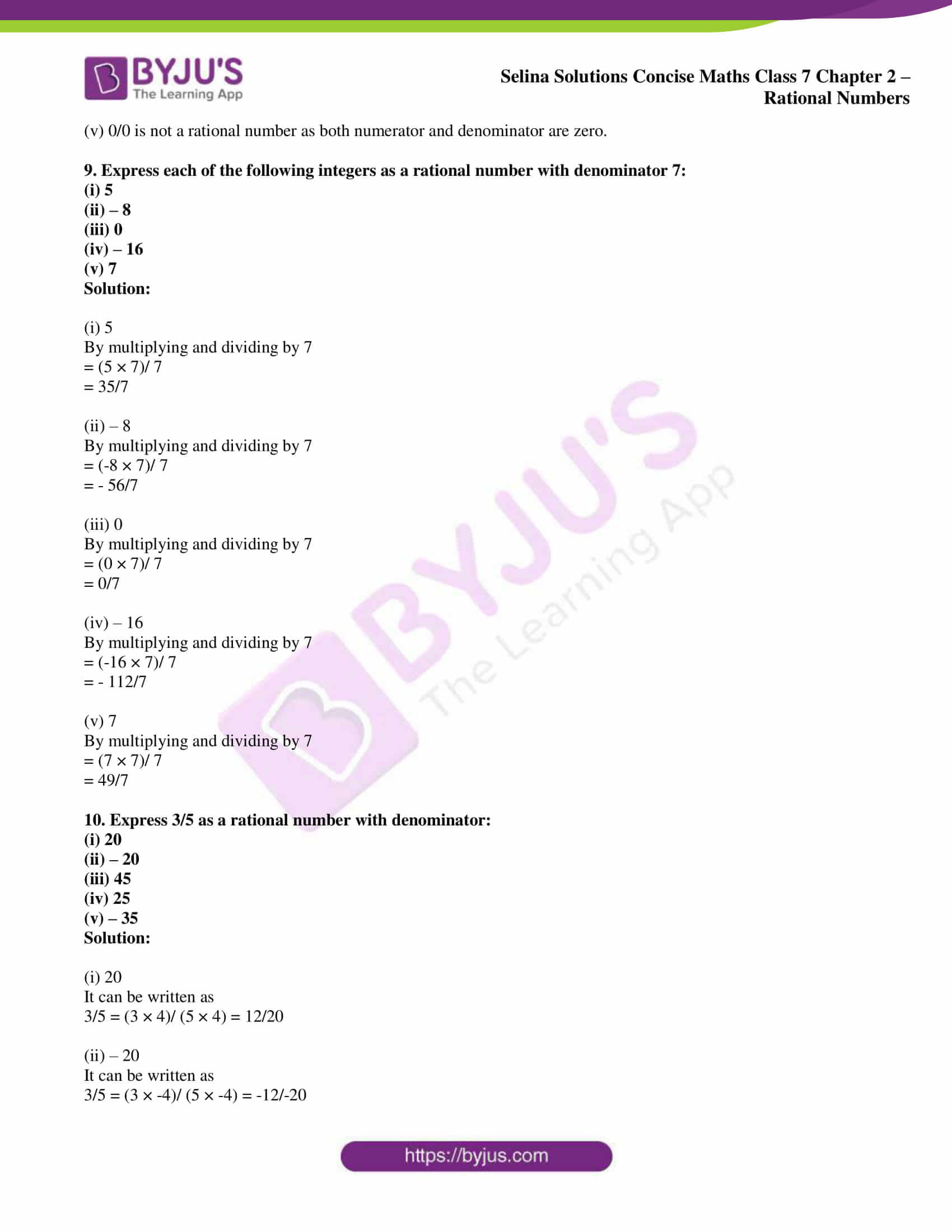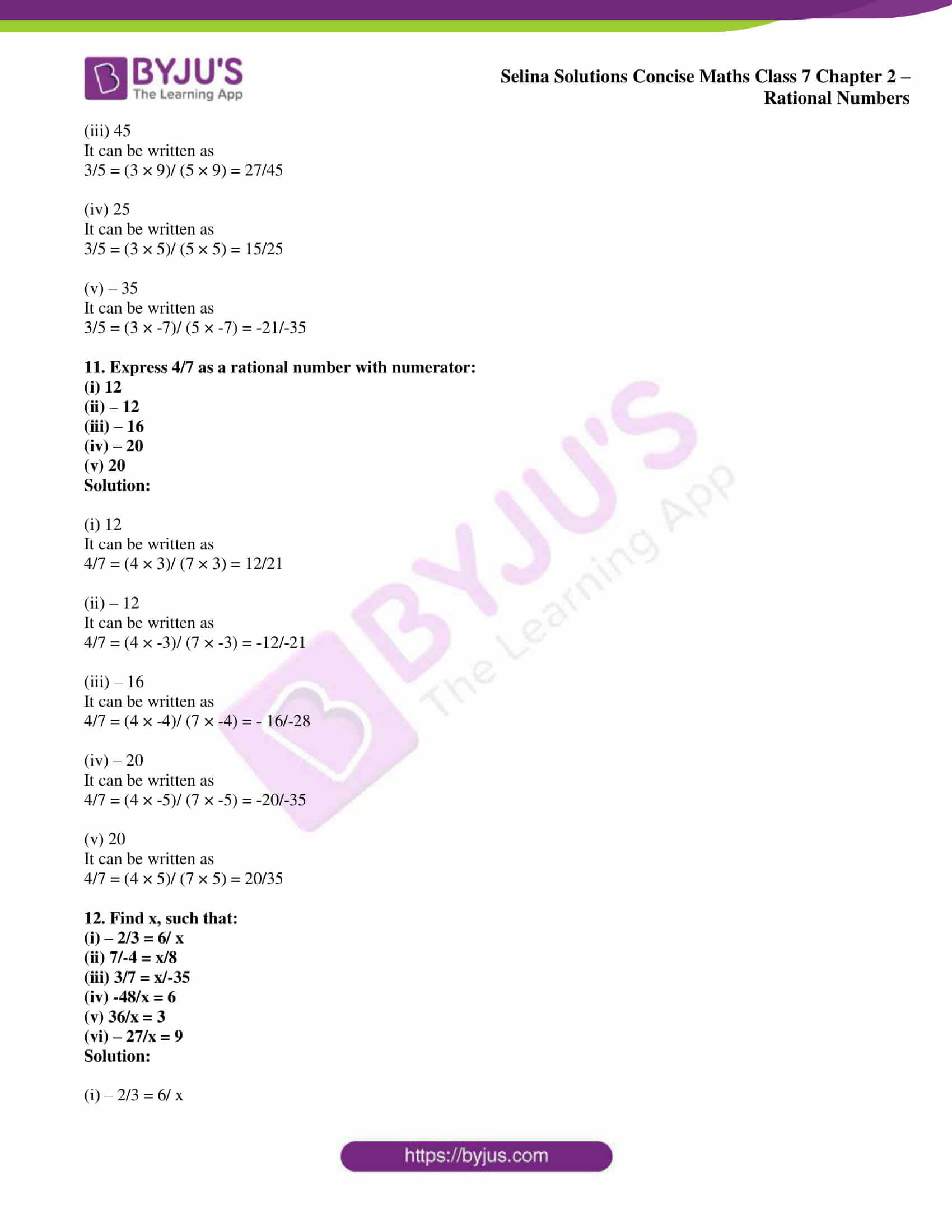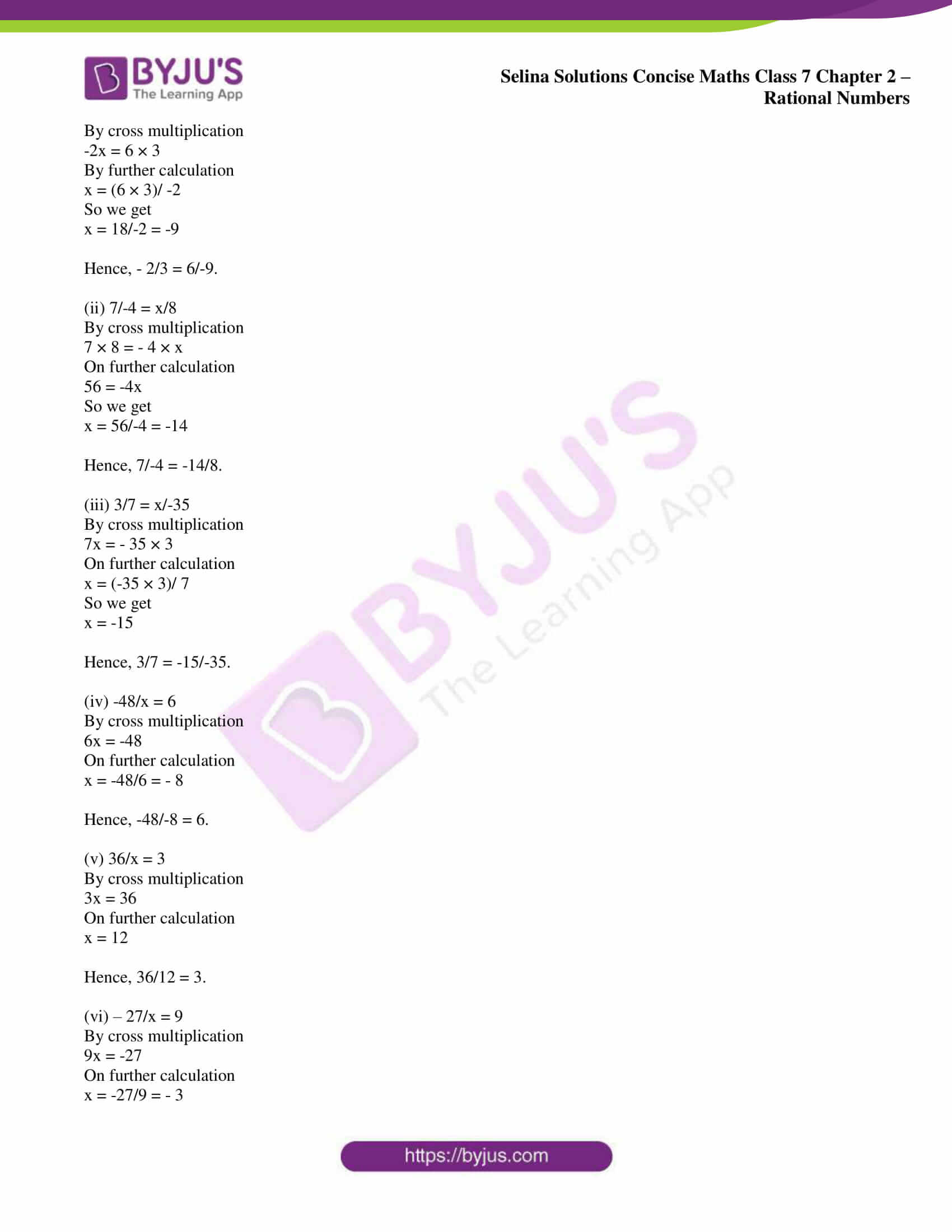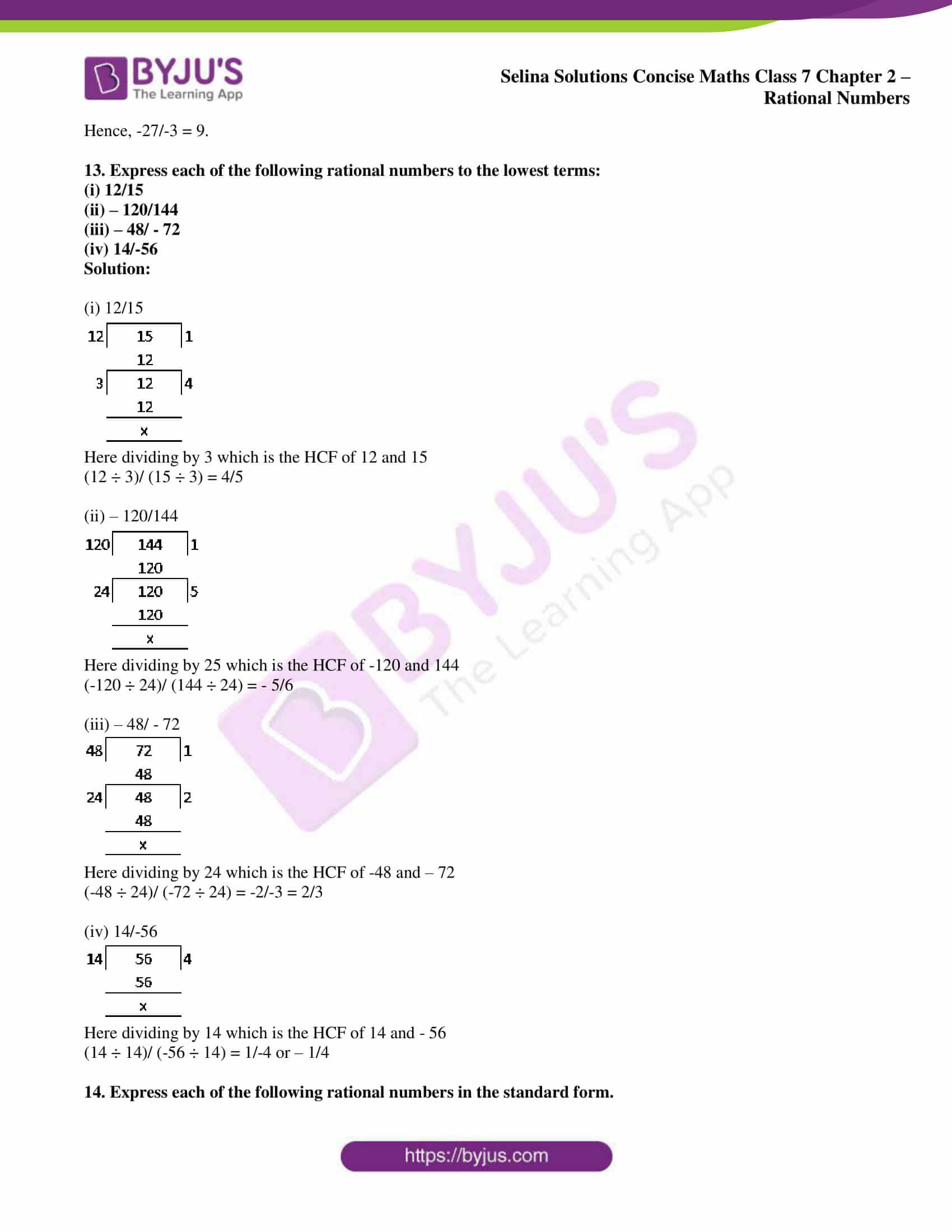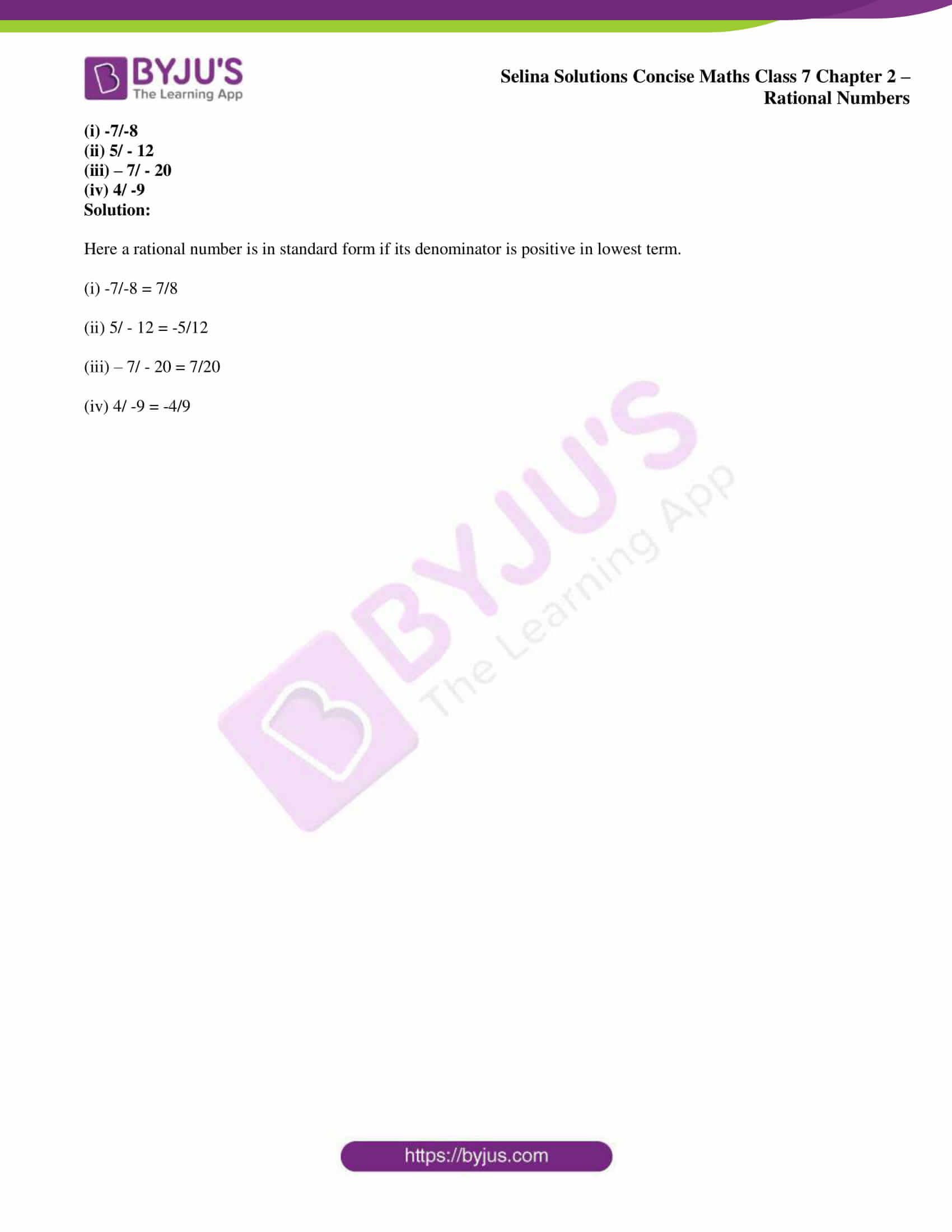### Access Selina Solutions Concise Maths Class 7 Chapter 2: Rational Numbers Exercise 2A

1. Write down a rational number whose numerator is the largest number of two digits and denominator is the smallest number of four digits.

Solution:

We know that the largest two digit number is 99

So the smallest four digit number is 1000

Numerator = 99

Denominator = 1000

Rational number = 99/1000

2. Write the numerator of each of the following rational numbers:

(i) – 125/127

(ii) 37/ -137

(iii) – 85/ 93

(iv) 2

(v) 0

Solution:

(i) – 125/127

Here the numerator = – 125

(ii) 37/ -137

Here the numerator = 37

(iii) – 85/ 93

Here the numerator = – 85

(iv) 2 = 2/1

Here the numerator = 2

(v) 0 = 0/1

Here the numerator = 0

3. Write the denominator of each of the following rational numbers:

(i) 7/ -15

(ii) – 18/29

(iii) – 3/4

(iv) – 7

(v) 0

Solution:

(i) 7/ -15

Here the denominator = – 15

(ii) – 18/29

Here the denominator = 29

(iii) – 3/4

Here the denominator = 4

(iv) – 7 = -7/1

Here the denominator = 1

(v) 0 = 0/1

Here the denominator = 1

4. Write down a rational number with numerator (-5) × (-4) and with denominator (28 – 27) × (8 – 5).

Solution:

It is given that

Numerator = (-5) × (-4) = 20

Denominator = (28 – 27) × (8 – 5) = 1 × 3 = 3

So the rational number = 20/3

5. (i) – 15/1 in integer form is …….

(ii) 23/-1 in integer form is ……..

(iii) If 18 = 18/a then a = …….

(iv) If – 57 = 57/a then a = ………

Solution:

(i) – 15/1 in integer form is – 15.

(ii) 23/-1 in integer form is – 23.

(iii) If 18 = 18/a then a = 18/18 = 1.

(iv) If – 57 = 57/a then a = 57/-57 = – 1.

6. Separate positive and negative rational numbers from the following:

-3/ 5, 3/-5, -3/-5, 3/5, 0, -13/-3, 15/-8, -15/8

Solution:

Here the positive rational numbers are

-3/-5 = 3/5 as both are negative

-13/-3 = 13/3 as both are negative and 3/5

Similarly the negative rational numbers are

-3/5, 3/ -5, 15/-8 and -15/8

0 is neither positive nor negative integer.

7. Find three rational numbers equivalent to

(i) 3/5

(ii) 4/-7

(iii) -5/9

(iv) 8/-15

Solution:

(i) 3/5

It can be written as

3/5 = (3 × 2)/ (5 × 2) = 6/10

3/5 = (3 × 3)/ (5 × 3) = 9/15

3/5 = (3 × 4)/ (5 × 4) = 12/20

Therefore, 6/10, 9/15 and 12/20 are the rational numbers which are equivalent to the given rational number 3/5.

(ii) 4/-7

It can be written as

4/-7 = (4 × 2)/ (-7 × 2) = 8/ -14

4/-7 = (4 × 3)/ (-7 × 3) = 12/-21

4/-7 = (4 × 4)/ (-7 × 4) = 16/ -28

Therefore, 8/-14, 12/-21 and 16/-28 are the rational numbers which are equivalent to the given rational number 4/-7.

(iii) -5/9

It can be written as

-5/9 = (-5 × 2)/ (9 × 2) = -10/18

-5/9 = (-5 × 3)/ (9 × 3) = – 15/27

-5/9 = (-5 × 4)/ (9 × 4) = -20/36

Therefore, -10/18, -15/27 and -20/36 are the rational numbers which are equivalent to the given rational number -5/9.

(iv) 8/-15

It can be written as

8/-15 = (8 × 2)/ (-15 × 2) = 16/-30

8/-15 = (8 × 3)/ (-15 × 3) = 24/ -45

8/-15 = (8 × 4)/ (-15 × 4) = 32/-60

Therefore, 16/-30, 24/-45 and 32/-60 are the rational numbers which are equivalent to the given rational number 8/-15.

8. Which of the following are not rational numbers:

(i) – 3

(ii) 0

(iii) 0/4

(iv) 8/0

(v) 0/0

Solution:

(i) – 3 = -3/1 is a rational number.

(ii) 0 = 0/1 is a rational number.

(iii) 0/4 is a rational number.

(iv) 8/0 is not a rational number.

(v) 0/0 is not a rational number as both numerator and denominator are zero.

9. Express each of the following integers as a rational number with denominator 7:

(i) 5

(ii) – 8

(iii) 0

(iv) – 16

(v) 7

Solution:

(i) 5

By multiplying and dividing by 7

= (5 × 7)/ 7

= 35/7

(ii) – 8

By multiplying and dividing by 7

= (-8 × 7)/ 7

= – 56/7

(iii) 0

By multiplying and dividing by 7

= (0 × 7)/ 7

= 0/7

(iv) – 16

By multiplying and dividing by 7

= (-16 × 7)/ 7

= – 112/7

(v) 7

By multiplying and dividing by 7

= (7 × 7)/ 7

= 49/7

10. Express 3/5 as a rational number with denominator:

(i) 20

(ii) – 20

(iii) 45

(iv) 25

(v) – 35

Solution:

(i) 20

It can be written as

3/5 = (3 × 4)/ (5 × 4) = 12/20

(ii) – 20

It can be written as

3/5 = (3 × -4)/ (5 × -4) = -12/-20

(iii) 45

It can be written as

3/5 = (3 × 9)/ (5 × 9) = 27/45

(iv) 25

It can be written as

3/5 = (3 × 5)/ (5 × 5) = 15/25

(v) – 35

It can be written as

3/5 = (3 × -7)/ (5 × -7) = -21/-35

11. Express 4/7 as a rational number with numerator:

(i) 12

(ii) – 12

(iii) – 16

(iv) – 20

(v) 20

Solution:

(i) 12

It can be written as

4/7 = (4 × 3)/ (7 × 3) = 12/21

(ii) – 12

It can be written as

4/7 = (4 × -3)/ (7 × -3) = -12/-21

(iii) – 16

It can be written as

4/7 = (4 × -4)/ (7 × -4) = – 16/-28

(iv) – 20

It can be written as

4/7 = (4 × -5)/ (7 × -5) = -20/-35

(v) 20

It can be written as

4/7 = (4 × 5)/ (7 × 5) = 20/35

12. Find x, such that:

(i) – 2/3 = 6/ x

(ii) 7/-4 = x/8

(iii) 3/7 = x/-35

(iv) -48/x = 6

(v) 36/x = 3

(vi) – 27/x = 9

Solution:

(i) – 2/3 = 6/ x

By cross multiplication

-2x = 6 × 3

By further calculation

x = (6 × 3)/ -2

So we get

x = 18/-2 = -9

Hence, – 2/3 = 6/-9.

(ii) 7/-4 = x/8

By cross multiplication

7 × 8 = – 4 × x

On further calculation

56 = -4x

So we get

x = 56/-4 = -14

Hence, 7/-4 = -14/8.

(iii) 3/7 = x/-35

By cross multiplication

7x = – 35 × 3

On further calculation

x = (-35 × 3)/ 7

So we get

x = -15

Hence, 3/7 = -15/-35.

(iv) -48/x = 6

By cross multiplication

6x = -48

On further calculation

x = -48/6 = – 8

Hence, -48/-8 = 6.

(v) 36/x = 3

By cross multiplication

3x = 36

On further calculation

x = 12

Hence, 36/12 = 3.

(vi) – 27/x = 9

By cross multiplication

9x = -27

On further calculation

x = -27/9 = – 3

Hence, -27/-3 = 9.

13. Express each of the following rational numbers to the lowest terms:

(i) 12/15

(ii) – 120/144

(iii) – 48/ – 72

(iv) 14/-56

Solution:

(i) 12/15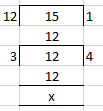Here dividing by 3 which is the HCF of 12 and 15

(12 ÷ 3)/ (15 ÷ 3) = 4/5

(ii) – 120/144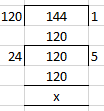Here dividing by 25 which is the HCF of -120 and 144

(-120 ÷ 24)/ (144 ÷ 24) = – 5/6

(iii) – 48/ – 72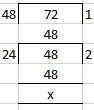Here dividing by 24 which is the HCF of -48 and – 72

(-48 ÷ 24)/ (-72 ÷ 24) = -2/-3 = 2/3

(iv) 14/-56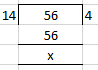Here dividing by 14 which is the HCF of 14 and – 56

(14 ÷ 14)/ (-56 ÷ 14) = 1/-4 or – 1/4

14. Express each of the following rational numbers in the standard form.

(i) -7/-8

(ii) 5/ – 12

(iii) – 7/ – 20

(iv) 4/ -9

Solution:

Here a rational number is in standard form if its denominator is positive in lowest term.

(i) -7/-8 = 7/8

(ii) 5/ – 12 = -5/12

(iii) – 7/ – 20 = 7/20

(iv) 4/ -9 = -4/9